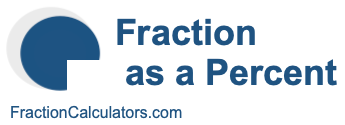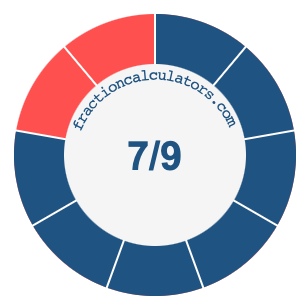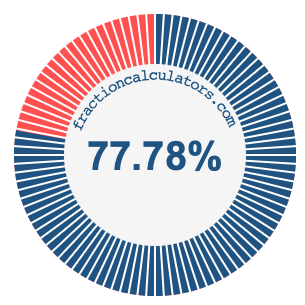7/9 as a percentWhat is 7/9 as a percent? Here we will explain and show you how to calculate and convert 7/9 to a percent.

In addition, we will also illustrate the fraction 7/9 on a pie chart and 7/9 as a percent on a pie chart.

7/9 is a fraction that means 7 out of 9, and percent means something out of 100. We can therefore make the following equation to get 7/9 as a percent:

 7 9
=
 percent 100

To find the percent in the equation above, we multiply 7 by 100 and then divide the product by 9. Here is the solution to the equation above and the answer to 7/9 as a percent:

 7 9
≈
 77.78 100

7/9 ≈ 77.78%

The illustrations below show you how 7/9 and 77.78% divide a pie chart differently, but cover the same area because they are the same.Fraction as a Percent Calculator
You can use this tool to convert another fraction to percent.

/

7/10 as a percent
Here is the next fraction on our list that we have converted to a percent for you.